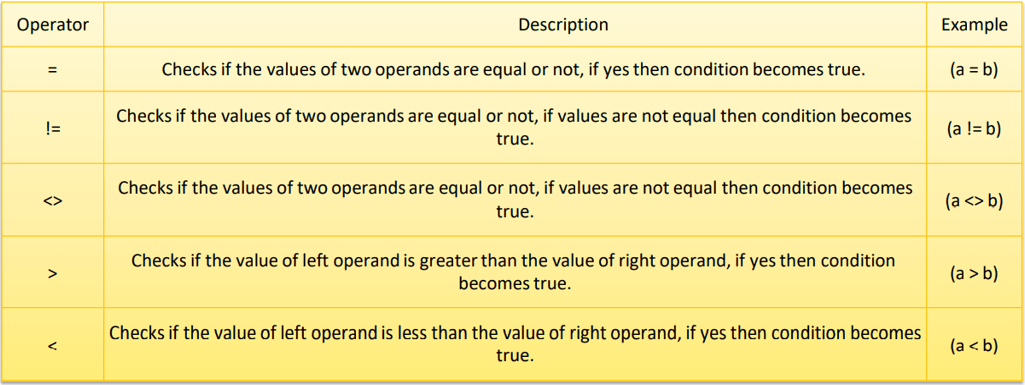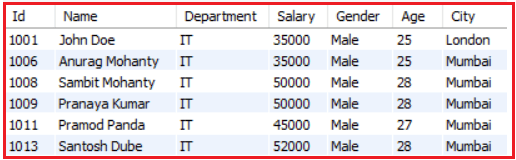# Comparison Operators in MySQL

## Comparison Operators in MySQL with Examples

In this article, I am going to discuss Comparison Operators in MySQL with Examples. Please read our previous article where we discussed Conditions in MySQL with Examples. At the end of this article, you will understand what are Comparison operators and why we need and the different types of Comparison operators available in MySQL with examples.

##### What are Comparison Operators in MySQL?

As the name suggests the Comparison Operators in MySQL are used to compare two values i.e. these operators are used for comparing one expression with another expression. The comparison operators determine whether the two values are equal or a value is greater than the other, or less than the other. The comparison operators can be applied to numbers, strings, and dates. The result of a comparison can be TRUE, FALSE, or NULL (When one or both the expression contains NULL values).

##### Types of Comparison Operators in MySQL

The different types of comparison operators that are available in MySQL Database are as follows:

1. Equal (=) Operator
2. Not Equal (!= or <>) Operator
3. Greater Than (>) Operator
4. Less Than (<) Operator
5. Greater Than or Equal To (>=) Operator
6. Less Than or Equal To (<=) Operator

The following table shows the list of all the comparison operators in SQL.##### Comparison Operators Examples in MySQL:

Let us understand how to use Comparison Operators in MySQL with Examples. We are going to use the following Employee table to understand the Comparison Operators.Please use the following SQL Script to create the company database and employee table with the required records.

```CREATE DATABASE company;
USE company;

CREATE TABLE employee (
Id INT PRIMARY KEY,
Name VARCHAR(45) NOT NULL,
Department VARCHAR(45) NOT NULL,
Salary FLOAT NOT NULL,
Gender VARCHAR(45) NOT NULL,
Age INT NOT NULL,
City VARCHAR(45) NOT NULL
);

INSERT INTO employee (Id, `Name`, Department, Salary, Gender, Age, City) VALUES (1001, 'John Doe', 'IT', 35000, 'Male', 25, 'London');
INSERT INTO employee (Id, `Name`, Department, Salary, Gender, Age, City) VALUES (1002, 'Mary Smith', 'HR', 45000, 'Female', 27, 'London');
INSERT INTO employee (Id, `Name`, Department, Salary, Gender, Age, City) VALUES (1003, 'James Brown', 'Finance', 50000, 'Male', 28, 'London');
INSERT INTO employee (Id, `Name`, Department, Salary, Gender, Age, City) VALUES (1004, 'Mike Walker', 'Finance', 50000, 'Male', 28, 'London');
INSERT INTO employee (Id, `Name`, Department, Salary, Gender, Age, City) VALUES (1005, 'Linda Jones', 'HR', 75000, 'Female', 26, 'London');
INSERT INTO employee (Id, `Name`, Department, Salary, Gender, Age, City) VALUES (1006, 'Anurag Mohanty', 'IT', 35000, 'Male', 25, 'Mumbai');
INSERT INTO employee (Id, `Name`, Department, Salary, Gender, Age, City) VALUES (1007, 'Priyanla Dewangan', 'HR', 45000, 'Female', 27, 'Mumbai');
INSERT INTO employee (Id, `Name`, Department, Salary, Gender, Age, City) VALUES (1008, 'Sambit Mohanty', 'IT', 50000, 'Male', 28, 'Mumbai');
INSERT INTO employee (Id, `Name`, Department, Salary, Gender, Age, City) VALUES (1009, 'Pranaya Kumar', 'IT', 50000, 'Male', 28, 'Mumbai');
INSERT INTO employee (Id, `Name`, Department, Salary, Gender, Age, City) VALUES (1010, 'Hina Sharma', 'HR', 75000, 'Female', 26, 'Mumbai');
INSERT INTO employee (Id, `Name`, Department, Salary, Gender, Age, City) VALUES (1011, 'Pramod Panda', 'IT', 45000, 'Male', 27, 'Mumbai');
INSERT INTO employee (Id, `Name`, Department, Salary, Gender, Age, City) VALUES (1012, 'Preety Tiwary', 'HR', 55000, 'Female', 28, 'Mumbai');
INSERT INTO employee (Id, `Name`, Department, Salary, Gender, Age, City) VALUES (1013, 'Santosh Dube', 'IT', 52000, 'Male', 28, 'Mumbai');
INSERT INTO employee (Id, `Name`, Department, Salary, Gender, Age, City) VALUES (1014, 'Sara Talour', 'HR', 85000, 'Female', 26, 'London');
INSERT INTO employee (Id, `Name`, Department, Salary, Gender, Age, City) VALUES (1015, 'Pamela Kar', 'Finance', 70000, 'Female', 26, 'London');
```
##### Equal (=) Comparison Operator in MySQL

The Equal (=) Operator is used to check whether the two expressions are equal or not. If both the expressions are equal then the condition becomes true and will return the matched rows. For example, the following SQL query will return all the records from the Employee table where the Department is IT. Here the expression is Department = ‘IT’ and it will check all the Department column values in the employee table and those values which are Matched with the value IT will be returned.

SELECT * FROM Employee WHERE Department = ‘IT’;

Once you execute the above SQL Statement, you will get the following result set which includes only the employees who belong to the IT Department.##### Not Equal (!=) Comparison Operator in MySQL

The Not Equal (!=) Operator is just the opposite of the equal operator. That means this operator is used to check whether the two expressions are equal or not. If both the expressions are not equal then the condition becomes true and will return the not-matched records. For example, the below SQL Statement will return all records from the Employee table except the employees whose department is IT.

SELECT * FROM Employee WHERE Department != ‘IT’;

Once you execute the above Select Statement, then you will get the following result set which includes all the employees except the IT Department employees.##### Not Equal (<>) Comparison Operator in MySQL

The Not Equal (<>) Operator is the same as the Not Equal (!=) operator in MySQL. That means it is also used to check whether the two expressions are equal or not. If both the expressions are not equal then the condition becomes true and will return the not-matched records. Let us take the same example as the previous one. In the below example, it will return all records from the Employee table except the employees whose department is IT.

SELECT * FROM Employee WHERE Department <> ‘IT’;

The above SQL Query will give the same output as the previous example as shown in the below image.Note: The != operator is not an ISO standard

##### Greater Than (>) Comparison Operator in MySQL

The Greater Than (>) Operator is used to check whether the left-hand expression value is higher than the right-hand expression value. If the left-hand expression value is higher than the right-hand expression value then the condition becomes true and it will return the matched records. For example, the below SQL query will return all records from the Employee table where the employee age is greater than 26.

SELECT * FROM Employee WHERE Age > 26;

Once you execute the above SQL query, you will get the following result set which includes all the employees whose age is greater than 26.##### Less Than (<) Comparison Operator in MySQL

The Less Than (>) Operator is used to check whether the left-hand expression value is lower than the right-hand expression value. If the left-hand expression value is lower than the right-hand expression value then the condition becomes true and will return the matched records. For example, the below SQL query will return all records from the Employee table where the employee age is less than 27.

SELECT * FROM Employee WHERE Age < 27;

Once you execute the above SQL Statement, you will get the following result set which includes all the employees whose age is less than 27.##### Greater Than or Equal To (>=) Operator in MySQL

The Greater than or Equal To (>=) Operator is used to check whether the left-hand expression value is higher than or equals to the right-hand expression value or not. If the left-hand expression value is higher than or equals to the right-hand expression value then the condition becomes true and will return all the matched records. For example, the following SQL Query will return all the records from the Employee table where the Salary is greater than or equal to 50000.

SELECT * FROM Employee WHERE Salary >= 50000;

When you execute the above SQL query, you will get the following result set which includes all the employees whose salary is greater than or equals 50000.##### Less Than or Equal To (<=) Operator in MySQL

The Less than or Equal To (<=) Operator is used to checking whether the left-hand expression value is lower than or equals to the right-hand expression value or not. If the left-hand expression value is lower than or equals to the right-hand expression value then the condition becomes true and it will return all the matching records. For example, the following SQL Query will return all the records from the Employee table where the Salary is less than or equal to 50000.

SELECT * FROM Employee WHERE Salary <= 50000;

When you execute the above SQL Statement, then you will get the following result set which includes all the employees whose salary is less than or equals 50000.In the next article, I am going to discuss the Logical AND, OR, NOT Operators in MySQL with Examples. Here, in this article, I try to explain Comparison Operators in MySQL with Examples. I hope you enjoy this article.